Courses

# RD Sharma Solutions Ex-1.2, Number System, Class 9, Maths Class 9 Notes | EduRev

## Class 9 : RD Sharma Solutions Ex-1.2, Number System, Class 9, Maths Class 9 Notes | EduRev

The document RD Sharma Solutions Ex-1.2, Number System, Class 9, Maths Class 9 Notes | EduRev is a part of the Class 9 Course RD Sharma Solutions for Class 9 Mathematics.
All you need of Class 9 at this link: Class 9

Q1. Express the following rational numbers as decimals:

(i) 42/100

(ii) 327/500

(iii) 15/4

Solution:

(i) By long division method

100)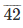( 0.42

400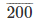200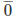Therefore,  42/100 = 0.42

(ii) By long division method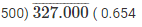3000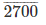2500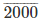2000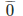Therefore, 327/500 = 0.654

(iii) By long division method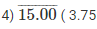12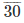28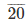20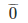Therefore, 15/4 = 3.75

Q2. Express the following rational numbers as decimals:

(i) 2/3

(ii)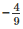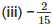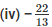(v) 437/999

Solution:

(i) By long division method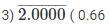18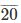18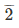Therefore, 2/3 = 0.66

(ii) By long division method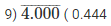3600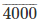36003600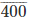Therefore,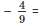– 0.444

(iii) By long division method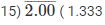15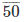454545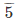Therefore, 2/15 = -1.333

(iv) By long division method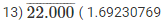13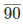78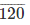117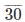26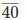39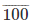91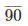78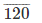117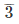Therefore,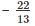= – 1.69230769

(v) By long division method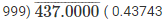3996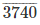2997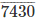6993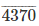3996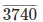2997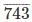Therefore, 437/999 = 0.43743

Q3. Look at several examples of rational numbers in the form of p/q (q ≠ 0), where p and q are integers with no common factor other than 1 and having terminating decimal representations. Can you guess what property q must satisfy?

Solution: A rational number p/q is a terminating decimal only, when prime factors of q are q and 5 only. Therefore, p/q  is a terminating decimal only, when prime factorization of q must have only powers of 2 or 5 or both.

Offer running on EduRev: Apply code STAYHOME200 to get INR 200 off on our premium plan EduRev Infinity!

91 docs

,

,

,

,

,

,

,

,

,

,

,

,

,

,

,

,

,

,

,

,

,

,

,

,

,

,

,

,

,

,

;